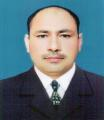# Center of Excellence in Fluid Dynamics

### Introduction

Due to the significant role in industry and engineering, it is very important to study the flow behavior of Newtonian, non-Newtonian and nanofluids. This analysis will be focused on the study of MHD flow of Newtonian, non-Newtonian and nanofluids in a porous medium with and without combined effects of heat and mass transfer. The customary models of Newtonian and non-Newtonian fluids via fractional calculus will be generalized. This reseaech will cover various flow regimes and their solutions, including, Newtonian, non-Newtonian and nanofluids via integral transforms and numerical schemes.

To become a leading centre of fluid dynamics in the region.

The mission of this CoE is o find the exact and numerical solutions for unsteady magnetohydrodynamic free convection/mixed convection flows of Newtonian and non-Newtonian fluids with heat and mass transfer.To generalize the classical models of Newtonian and non-Newtonian fluids via fractional calculus. To discuss the important issues regarding the nanofluid motion and heat transfer analysis in perspective of the obtained results. To obtain the exact analytical solutions using the integral transform techniques. The results will be published in high rank world prestigeous journals.

## Head of Center

### FARHAD ALI## MembersNADEEM AHMAD SHEIKHMADEHA GOHARAAMINA